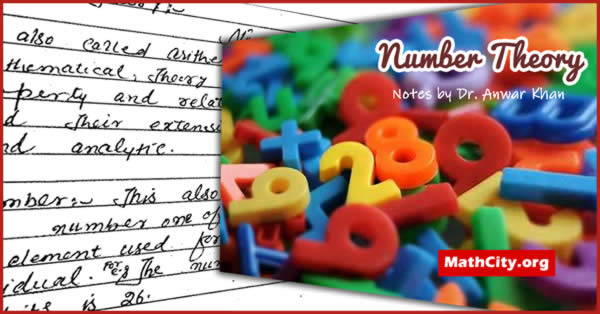# Number Theory Notes by Anwar KhanMathematicians who specialize in number theory examine the characteristics and connections between integers. “Higher arithmetic” and “the queen of mathematics” are some names for it. Because it examines the characteristics and connections between integers and arithmetic functions, number theory is interesting. It has numerous uses in coding theory, combinatorics, cryptography, and other branches of mathematics. Like the ones discussed in this article, it too has a tonne of amazing information and algorithms.

These notes are provided by Mr. Anwar Khan. We are very thankful to him for providing these notes.

 Name Number Theory Notes Dr. Anwar Khan 219 pages Handwritten images in PDF 4.5 mB
• Number Theory
• Divisibility
• Euclid’s Theorem
• Common Division
• Method of finding great common divisor
• G.C.D. more than two integers
• Least Common Multiple
• The Linear Diophantine Equation
• Theory of Primes
• Composite Number
• Prime Divisor
• Congruence
• Complete Residue System
• Solutions of the Congruence
• Perfect Number
• Reduce residue System